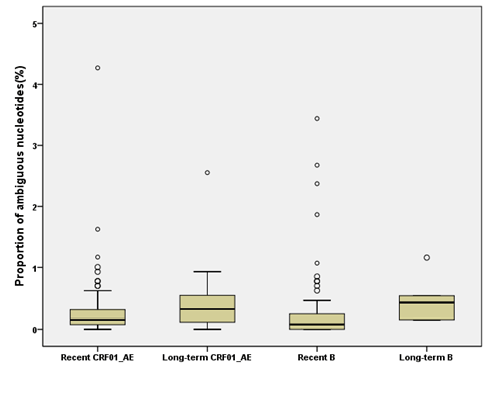Figure 1: Comparison of proportions of ambiguous nucleotides between recent and long-term infections. The ends of the whiskers mark the 5th and 95th percentile. Outliers are marked by dots. For subtype CRF01_AE, the median value of ambiguous nucleotides fraction for recent and long-term infections were 0.155% and 0.318%, respectively, P=0.031. For subtype B, the median value of ambiguous nucleotides fraction for recent and long-time infections were 0.080% and 0.425% respectively, P=0.02.
Goto home»